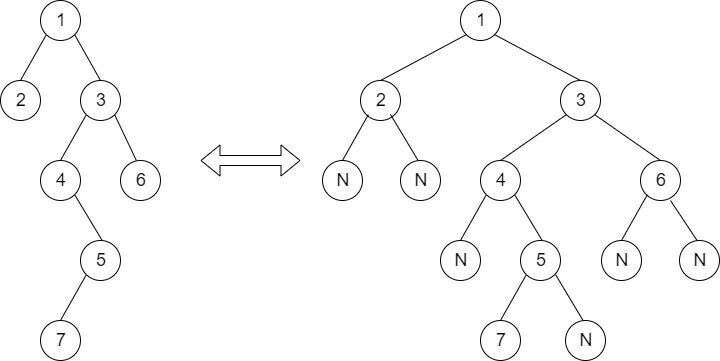Kth Ancestor in a Tree
Easy Accuracy: 34.95% Submissions: 7977 Points: 2

Given a binary tree of size  N, a node and a positive integer K., your task is to complete the function kthAncestor(), the function should return the Kth ancestor of the given node in the binary tree. If there does not exist any such ancestor then return -1.
Example:Input:
K = 2
Node = 4
Output: 1
Since, K is 2 and node is 4, so we
first need to locate the node and
look k times its ancestors.
Here in this Case node 4 has 1 as his
2nd Ancestor aka the Root of the tree.


Input:
First line of input contains the number of test cases T. For each test case, there will be only a single line of input which is a string representing the tree as described below:

1. The values in the string are in the order of level order traversal of the tree where, numbers denote node values, and a character “N” denotes NULL child.

2. For example:For the above tree, the string will be: 1 2 3 N N 4 6 N 5 N N 7 N

Output:
For each test the function should return the Kth ancestor of the given Node from the binary tree.
Constraints:
1<=T<=30
1<=N<=104
1<= K <= 100
Example:
Input:

1 3
1 2 3
2 4
1 2 3 4 5
Output:
1
1
Explanation:
Test Case 1: Given Tree is
1
/       \
2         3
K = 1 , given node = 3 , Kth ancestor of 3 is 1.